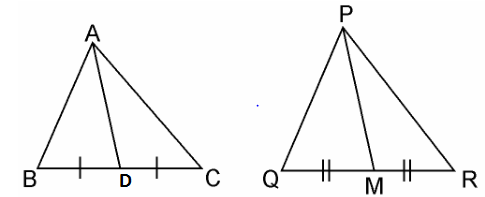# Diagonals of a trapezium ABCD with AB || DC intersect each other at the point O. If AB = 2CD, find the ratio of the areas of triangles AOB and COD.

Given

Diagonals of a trapezium ABCD with AB || DC intersect each other at the point O.

AB = 2CD

Find out

We have to find the ratio of the areas of triangles AOB and COD.

SolutionFrom ΔAOB and ΔCOD, we have

∠1 = ∠2 (Alternate angles)

∠3 = ∠4 (Alternate angles)

∠5 = ∠6 (Vertically opposite angle)

∴ ΔAOB ~ ΔCOD [AAA similarity criterion]

As we know, If two triangles are similar then the ratio of their areas are equal to the square of the ratio of their corresponding sides. Therefore,

Area of (ΔAOB)/Area of (ΔCOD) = AB2/CD2

= (2CD)2/CD2 [∴ AB = 2CD]

∴ Area of (ΔAOB)/Area of (ΔCOD)

= 4CD2/CD2 = 4/1

Therefore, the required ratio of the area of ΔAOB and ΔCOD = 4:1